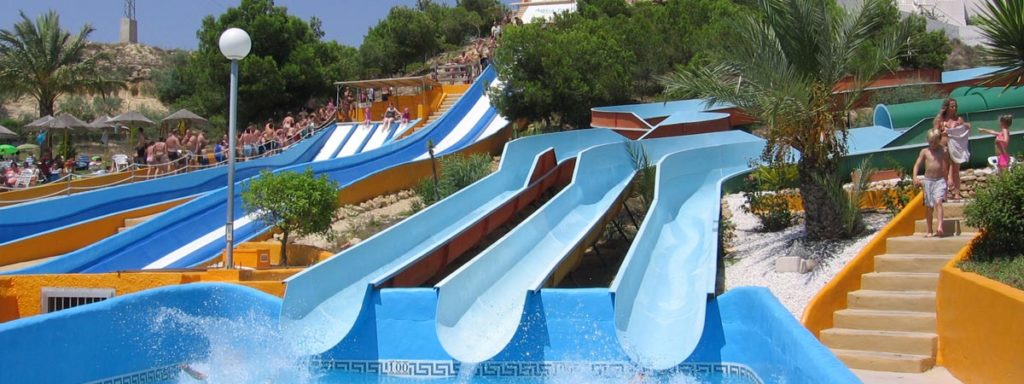Call +34 966 182 344 | +34 670 228 470

# Rojales

<p style="box-sizing: border-box; margin: 0px 0px 12px; color: rgb(74, 82, 93); font-family: " lucida="" grande",="" “lucida=”" sans="" unicode",="" sans",="" lucida,="" helvetica,="" arial,="" sans-serif;="" font-size:="" 15px;="" font-variant-numeric:="" normal;="" font-variant-east-asian:="" normal;“=”"><span lucida="" grande",“lucida=”" sans="" unicode",“lucida=”" sans",lucida,helvetica,arial,sans-serif;="" font-size:="" 12px;="" font-style:="" normal;="" font-variant:="" font-weight:="" 400;="" letter-spacing:="" orphans:="" 2;="" text-align:="" left;="" text-decoration:="" none;="" text-indent:="" 0px;="" text-transform:="" -webkit-text-stroke-width:="" white-space:="" word-spacing:="" 0px;“=”" style="box-sizing: border-box; color: rgb(51, 51, 51); font-family: " grande",="" “lucida=”" unicode",="" sans",="" lucida,="" helvetica,="" arial,="" sans-serif;="" background-color:="" rgb(255,="" 255,="" 255);="" float:="" display:="" inline="" !important;“=”"><span style="color: rgb(51, 51, 51); font-family: " lucida="" grande",="" “lucida=”" sans="" unicode",="" sans",="" lucida,="" helvetica,="" arial,="" sans-serif;="" font-size:="" 12px;="" background-color:="" rgb(255,="" 255,="" 255);“=”“>
”box-sizing: border-box; margin: 0px 0px 12px; color: rgb(74, 82, 93); font-family: " lucida="" grande",="" “lucida=”" sans="" unicode",="" sans",="" lucida,="" helvetica,="" arial,="" sans-serif;="" font-size:="" 15px;="" font-variant-numeric:="" normal;="" font-variant-east-asian:="" normal;“=”“>Rojalas is 35 minutes from Alicante airport along the N332 road, and is inland from the beautiful coastal area of Guardemar Del Sugura in Spain’s sunny Costa Blanca. Rojales is a very pretty and attractive town and has the river Segura running through it, the stunning beaches of Guardamar Del Sugura are only 10 minutes away and Torrevieja city is only 15 minutes. It is close to Ciudad Quesada, Benijofer and Almoradi. Rojales was originally a Moorish town built during the Moors occupation of Spain from the 8th to 13th century AD. There is lots of evidence of its historical past everywhere you look.

<span none;=”" 0px;="" 2;="" normal;="" 400;="" varela="" round",“helvetica=”" 14px;="" neue",helvetica,arial,sans-serif;="" left;="" 0px;“=”" style="box-sizing: border-box; color: rgb(74, 82, 93); font-family: " lucida="" grande",="" “lucida=”" sans="" unicode",="" sans",="" lucida,="" helvetica,="" arial,="" sans-serif;="" font-size:="" 12px;="" font-variant-numeric:="" font-variant-east-asian:="" display:="" inline;="" float:="" none;“=”"><span lucida="" grande",“lucida=”" sans="" unicode",“lucida=”" sans",lucida,helvetica,arial,sans-serif;="" font-size:="" 12px;="" font-style:="" normal;="" font-variant:="" font-weight:="" 400;="" letter-spacing:="" orphans:="" 2;="" text-align:="" left;="" text-decoration:="" none;="" text-indent:="" 0px;="" text-transform:="" -webkit-text-stroke-width:="" white-space:="" word-spacing:="" 0px;“=”" style="box-sizing: border-box; color: rgb(51, 51, 51); font-family: " grande",="" “lucida=”" unicode",="" sans",="" lucida,="" helvetica,="" arial,="" sans-serif;="" background-color:="" rgb(255,="" 255,="" 255);="" float:="" display:="" inline="" !important;“=”"><span style="color: rgb(51, 51, 51); font-family: " lucida="" grande",="" “lucida=”" sans="" unicode",="" sans",="" lucida,="" helvetica,="" arial,="" sans-serif;="" font-size:="" 12px;="" background-color:="" rgb(255,="" 255,="" 255);“=”“>
”box-sizing: border-box; margin: 0px 0px 12px; color: rgb(74, 82, 93); font-family: " lucida="" grande",="" “lucida=”" sans="" unicode",="" sans",="" lucida,="" helvetica,="" arial,="" sans-serif;="" font-size:="" 15px;="" font-variant-numeric:="" normal;="" font-variant-east-asian:="" normal;“=”">The surrounding environment is mostly agricultural, with a wide selection of fresh fruit such as Almonds, Oranges and Lemons as well as vegetables including artichokes, cauliflower and broccoli. Outside of the town there are also the traditional cave homes that are a feature of this area of Spain and are an amazing sight. No, Sunworld Estates do not sell Cave houses, sorry!!! Some of these homes are still lived in today and there are strict guidelines living there as there is a ban on electricity being used within them. Many of them will have an outhouse built nearby with an electricity supply which will be used for cooking and cleaning and the caves are used to sleep in. The caves are ideal in this climate as they maintain a constant temperature of approx. 22 degrees all year round thus protecting from the intense heat of the summer months but still remaining warm enough during the cooler winter months.

<span lucida="" grande",“lucida=”" sans="" unicode",“lucida=”" sans",lucida,helvetica,arial,sans-serif;="" font-size:="" 12px;="" font-style:="" normal;="" font-variant:="" font-weight:="" 400;="" letter-spacing:="" orphans:="" 2;="" text-align:="" left;="" text-decoration:="" none;="" text-indent:="" 0px;="" text-transform:="" -webkit-text-stroke-width:="" white-space:="" word-spacing:="" 0px;“=”" style="box-sizing: border-box; color: rgb(51, 51, 51); font-family: " grande",="" “lucida=”" unicode",="" sans",="" lucida,="" helvetica,="" arial,="" sans-serif;="" background-color:="" rgb(255,="" 255,="" 255);="" float:="" display:="" inline="" !important;“=”"><span style="color: rgb(51, 51, 51); font-family: " lucida="" grande",="" “lucida=”" sans="" unicode",="" sans",="" lucida,="" helvetica,="" arial,="" sans-serif;="" font-size:="" 12px;="" background-color:="" rgb(255,="" 255,="" 255);“=”“>
”box-sizing: border-box; margin: 0px 0px 12px; color: rgb(74, 82, 93); font-family: " lucida="" grande",="" “lucida=”" sans="" unicode",="" sans",="" lucida,="" helvetica,="" arial,="" sans-serif;="" font-size:="" 15px;="" font-variant-numeric:="" normal;="" font-variant-east-asian:="" normal;“=”“>The town of Rojales is typically Spanish with a lovely plaza at its centre which has cafes, restaurants and bars from where you can watch the world go by. There is a also a very pretty church and a museum which you can explore while visiting. It has banks and supermarkets as well as hairdressers and beauticians. Market day in Rojales is on Thursdays where it is situated along the river through the town. It is a very interesting and nice day out and you might always catch a bargain!! One very popular restaurant in Rojales is La Perla Roja (The Red Pearl) with its European and Spanish style cuisine, the food is consistently high quality and the prices are very reasonable. Booking can be necessary particularly during the busier months as it is quite small with no outdoor seating. If you have a Pizza lover in the family there’s nowhere better than Pizzeria 222 which has beautiful home cooked Pizzas and a selection of fresh Italian dishes. One for all the family.

<span none;=”" 0px;="" 2;="" normal;="" 400;="" varela="" round",“helvetica=”" 14px;="" neue",helvetica,arial,sans-serif;="" left;="" 0px;“=”" style="box-sizing: border-box; color: rgb(74, 82, 93); font-family: " lucida="" grande",="" “lucida=”" sans="" unicode",="" sans",="" lucida,="" helvetica,="" arial,="" sans-serif;="" font-size:="" 12px;="" font-variant-numeric:="" font-variant-east-asian:="" display:="" inline;="" float:="" none;“=”"><span lucida="" grande",“lucida=”" sans="" unicode",“lucida=”" sans",lucida,helvetica,arial,sans-serif;="" font-size:="" 12px;="" font-style:="" normal;="" font-variant:="" font-weight:="" 400;="" letter-spacing:="" orphans:="" 2;="" text-align:="" left;="" text-decoration:="" none;="" text-indent:="" 0px;="" text-transform:="" -webkit-text-stroke-width:="" white-space:="" word-spacing:="" 0px;“=”" style="box-sizing: border-box; color: rgb(51, 51, 51); font-family: " grande",="" “lucida=”" unicode",="" sans",="" lucida,="" helvetica,="" arial,="" sans-serif;="" background-color:="" rgb(255,="" 255,="" 255);="" float:="" display:="" inline="" !important;“=”"><span style="color: rgb(51, 51, 51); font-family: " lucida="" grande",="" “lucida=”" sans="" unicode",="" sans",="" lucida,="" helvetica,="" arial,="" sans-serif;="" font-size:="" 12px;="" background-color:="" rgb(255,="" 255,="" 255);“=”“>
”box-sizing: border-box; margin: 0px 0px 12px; color: rgb(74, 82, 93); font-family: " lucida="" grande",="" “lucida=”" sans="" unicode",="" sans",="" lucida,="" helvetica,="" arial,="" sans-serif;="" font-size:="" 15px;="" font-variant-numeric:="" normal;="" font-variant-east-asian:="" normal;“=”">New Builds are the most popular property sales in the general Rojales region with some super value new developments hitting the market this year.